# Local variations, method of

(diff) ← Older revision | Latest revision (diff) | Newer revision → (diff)

A direct method for numerically solving problems of optimal control with constraints on the phase coordinates and control functions, based on variation in the state space (see ).

In the method of local variations the original problem of optimal control, given as a Lagrange problem, is made discrete with respect to the argumentand the phase vector. The original problem is thus replaced by the problem of minimizing the additive functional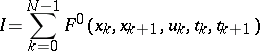(1)

under the constraints(2)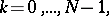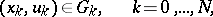(3)

whereandare the vectors of the phase coordinates and controls at the node(having dimensionsand, respectively),are given domains in the-dimensional space (and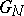describe the boundary conditions), andis the step of the partition of the original intervalfor the independent variable. An essential condition for the method of local variations is that the dimensionsandofandare equal, in which case the construction of an elementary operation turns out to be quite simple. An elementary operation is the determination of a control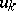that takes the system from the point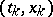to a neighbouring point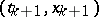. If the dimensions ofandare equal, and under certain additional constraints, the controlis defined on every interval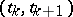by the solution of the system ofequations (2), obtained as a result of a finite-difference approximation of the system of differential equations of the original variational problem.

Suppose that for the initial approximation there is specified a polygonal curve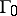: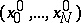for which (2) and (3) are satisfied. The algorithm of the method of local variations consists in successively improving the position of the nodes through whichpasses, realized as a result of successive local variation of each-th component of the vector. On each part from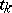to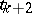,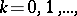for fixed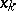andeach-th component ofin turn is varied with step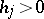. If as a result of this variation the value of the functional (1) decreases (with (2) and (3) satisfied), then a similar variation is carried out with the next-st component of, otherwise the-th component is varied with step. This local variation is carried out successively for all the nodes of. As a result, at the instant of ending the iteration one obtains a new polygonal curveon which the functional (1) takes a value not greater than on the initial approximation. Successive iterations are carried out similarly. If necessary one decreases the steps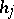and. The approximate solution of the original variational problem is determined by interpolation with respect to the values of the control found at each step.

For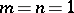the solution obtained by the method of local variations for given values ofandsatisfies the finite-difference approximation of the Euler equation up to terms of order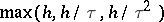(see ).

If the variation of the original polygonal curveis not restricted to successive variation of its nodes, and is carried out on a more complete graph corresponding to the chosen stepsandand including, one talks of the travelling-tube method.

For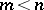, performing an elementary operation presents certain difficulties. In this case instead of the method of local variations one can use the travelling-wave method, which is close to it.

The method of local variations can be directly generalized to variational problems with non-additive functionals, in which the constraints have the character of isoperimetric conditions (see ). The method of local variations can be extended to variational problems in which the unknown functions depend on several independent variables and the corresponding functionals are given in the form of integrals over domains of different dimensions (see ).

How to Cite This Entry:
Local variations, method of. Encyclopedia of Mathematics. URL: http://encyclopediaofmath.org/index.php?title=Local_variations,_method_of&oldid=12292
This article was adapted from an original article by I.B. Vapnyarskii (originator), which appeared in Encyclopedia of Mathematics - ISBN 1402006098. See original article